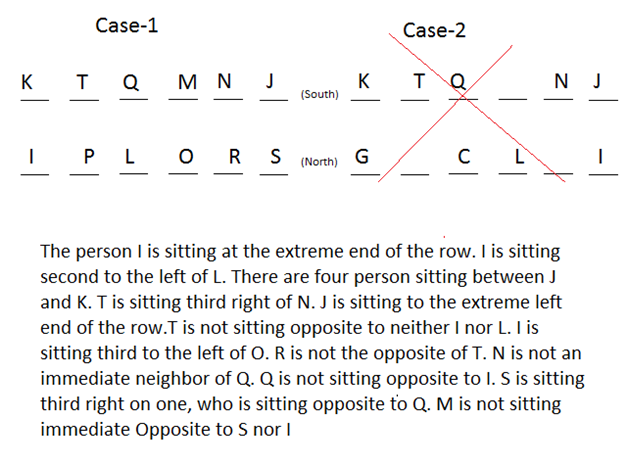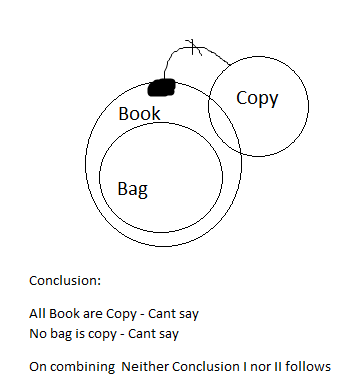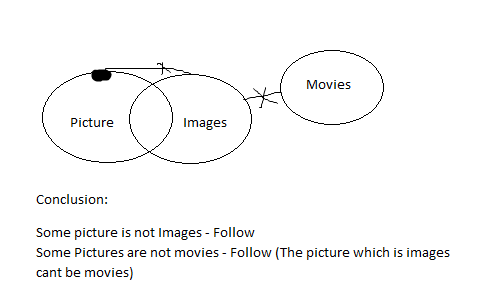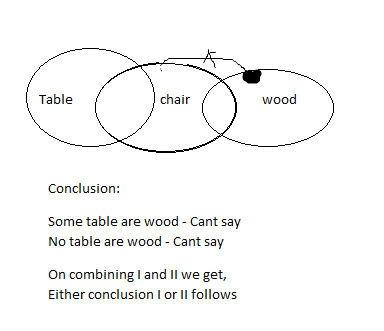# Reasoning Ability Quiz: 16 March 2021

Reasoning Ability Quiz: 16 March 2021 Published by: Anmol Bhatnagar Updated Tue, 16 Mar 2021 03:15 AM ISTSource: safalta.com
Q:  1-5) Direction: Read the following information carefully and answer the question given below.

Twelve people i.e. I, J, K, L, M, N, O, P, Q, R, S, and Tare sitting in two parallel rows containing six person each, in such a way that there is equal distance between adjacent people. In row 1 the person who are facing south are K, T, Q, M, N, and J. In row 2 the person who are facing north are I, P, L, O, R, and S but not necessarily in the same order.

### Free Demo Classes

The person I is sitting at the extreme end of the row. I is sitting second to the left of L. There are four person sitting between J and K. T is sitting third right of N. J is sitting to the extreme left end of the row.T is not sitting opposite to neither I nor L. I is sitting third to the left of O. R is not the opposite of T. N is not an immediate neighbor of Q. Q is not sitting opposite to I. S is sitting third right on one, who is sitting opposite to Q. M is not sitting immediate Opposite to S nor I.

Q-1) How many person sits between R and L ?
A) ONE
B) TWO
C)THREE
D)None of these

Q-2) Who sits in the front of I ?
A) K
B) T
C) Q
D) M

Q-3) Four of the following five are alike in a certain way and hence form a group. Which is the odd that does not belong to group?
A) K
B) J
C) I
D) S
E) L

Q-4) Who sits second to the right of O ?
A) P
B) S
C) R
D) L

Q-5) Four of the following five are alike in a certain way and hence form a group. Which is the odd that does not belong to a group?
A) M
B) J
C) L
D) Q
E) T

Q-6) In the following question below some statements are given followed by some conclusions. Taking the given statements to be true even if they seem to be at variance from commonly known facts, read the entire conclusion and then decide which of the given conclusion logically follows in the given statement.

Statement:
All Bag are Book
Only a few Book are Copy
Conclusion:
Some Bag can be copy
No bag is copy

1. Only conclusion I follows
2. Only conclusion II follows
3. Either conclusion I or II follows
4. Neither Conclusion I nor II follows
5. Both Conclusion  I and II follows

Q-7) In the following question below some statements are given followed by some conclusions. Taking the given statements to be true even if they seem to be at variance from commonly known facts, read the entire conclusion and then decide which of the given conclusion logically follows in the given statement.

Statement:
Only a Few Pictures are images
No images are Movie
Conclusion:
Some picture is not Images
Some Pictures are not movies
1. Only conclusion I follows
2. Only conclusion II follows
3. Either conclusion I or II follows
4. Neither Conclusion I nor II follows
5. Both Conclusion  I and II follows
Q-8) In the following question below some statements are given followed by some conclusions. Taking the given statements to be true even if they seem to be at variance from commonly known facts, read the entire conclusion and then decide which of the given conclusion logically follows in the given statement.

Statement:
Some table is chair
only a few woods are chair
Conclusion:
Some table are wood
No table are wood

Q: 9-10)Direction: Read the following information carefully and answer the question given below.

Q  4  R  \$  A  7  @  N  &  O  G  4  J  #  6  T  F  R  \$  &  R  7  %  L  5  !  ?  X

Q-9)How many symbols are there in the above arrangement, each of which is immediately preceded by a number and followed by symbol?
NONE
ONE
TWO
THREE
FOUR

Q-10) Which of the following is exactly in the middle between the seventh element from the right end the twelfth element from the left end in the above arrangement?

T
F
R
\$
&

Solution:Q-1) A
Q-2) A
Q-3) E
Q-4) B
Q-5) C

Q-6) BQ-7)Q-8)Q-9)
ANS – ONE
NUMBER – SYMBOL – SYMBOL
5  !  ?

Q-10)
Ans- F
Seven element from the right end – 7
element from the left end – 2
Hence the middle element is – F

### Disclaimer

अपनी वेबसाइट पर हम डाटा संग्रह टूल्स, जैसे की कुकीज के माध्यम से आपकी जानकारी एकत्र करते हैं ताकि आपको बेहतर अनुभव प्रदान कर सकें, वेबसाइट के ट्रैफिक का विश्लेषण कर सकें, कॉन्टेंट व्यक्तिगत तरीके से पेश कर सकें और हमारे पार्टनर्स, जैसे की Google, और सोशल मीडिया साइट्स, जैसे की Facebook, के साथ लक्षित विज्ञापन पेश करने के लिए उपयोग कर सकें। साथ ही, अगर आप साइन-अप करते हैं, तो हम आपका ईमेल पता, फोन नंबर और अन्य विवरण पूरी तरह सुरक्षित तरीके से स्टोर करते हैं। आप कुकीज नीति पृष्ठ से अपनी कुकीज हटा सकते है और रजिस्टर्ड यूजर अपने प्रोफाइल पेज से अपना व्यक्तिगत डाटा हटा या एक्सपोर्ट कर सकते हैं। हमारी Cookies Policy, Privacy Policy और Terms & Conditions के बारे में पढ़ें और अपनी सहमति देने के लिए Agree पर क्लिक करें।

Agree

Get free counselling session